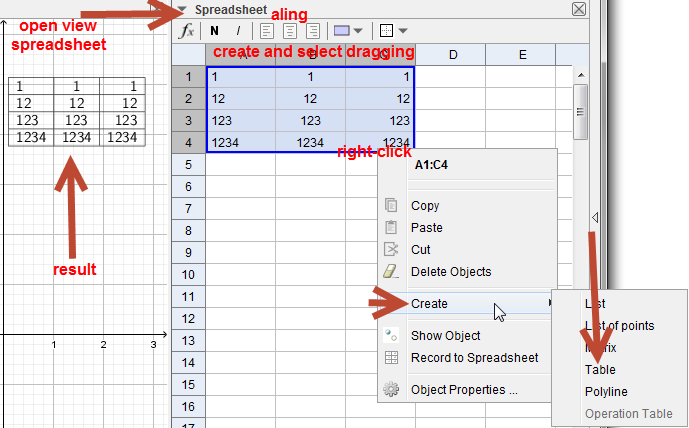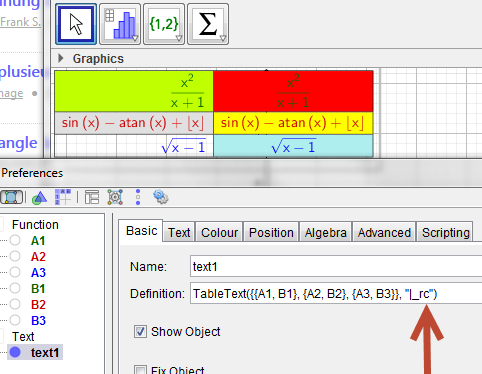# How to align to the right in LaTex formula?

arikheilig shared this question 5 years ago1

use tabletext()

see the help1

doesn't work in the LaTex text when using the "formula" option...1

I don't understand what you mean.

A single string of text is already aligned where you place it :D

And by the way, you should enter it as \text{blah blah blah}

As mathmagic said, in order to create nice LaTeX texts, use TableText command.

Check the related wiki page, that contains a lot of examples, to understand the syntax of the command.1

do you mean this?

Files: foro.ggb1

or this?

Files: foro.ggb1

this is what I meant in order to align texts/formulas to the right{r}, left {l}or center{c}

thank you for those who answerd

\begin{array}{r}

1\\

12\\

123\\

1234\\

\end{array}

\begin{array}{l}

1\\

12\\

123\\

1234\\

\end{array}

\begin{array}{c}

1\\

12\\

123\\

1234\\

\end{array}1

is this enough?1

editing the tabletext()1

same principle as solution of mathmagic (with correction vector)

but create vector in script (no duplicate objects)